# IBPS RRB 2018 Prelims : Quants – Day 5

Dear Bankersdaily Aspirants,

IBPS RRB PO & Office Assistant 2018 Preliminary Examination starts from 11th August, 2018. The IBPS RRB Exam 2018 is one of the most expected exam of the year. The reason for the consummate expectation is different from that of the others.

The number of candidates attending the IBPS RRB PO & Office Assistant is high when compared to the other exams like SBI & Insurance Exams. The other important factor is that , no English section is there in the preliminary examination of the IBPS RRB PO & Assistant Exams. This is really a boost to the candidates as many of the aspirants couldn’t get the requisite marks in the English section and this exam is the best bet for the aspirants.

Since only few days are left for the IBPS RRB PO & Assistant Exam 2018 Preliminary Exam , we will be providing daily 20 Questions in Quantitative Aptitude & Reasoning Section to evoke the preparation mode.

Aspirants can answer the Questions by commenting the answers for the Questions in the comments section. We will be providing the answers for the Questions @ 6:00 P.M everyday.

## IBPS RRB PO & Assistant 2018 – Prelims Exam – Day 5

Topic : Quantitative Aptitude

Total Number of Questions : 20

Total Time : 15 minutes

D.1-3) Study the following information and answer the given questions:

The table shows the number of two wheelers and four wheelers manufactured and sold 5 different companies.

 Company Number of two wheelers manufactured Number of two wheelers sold Number of four wheelers manufactured Number of four wheelers sold P 900 560 540 370 Q 1020 600 450 290 R 850 430 420 240 S 980 420 500 330 T 1010 560 660 220

Q.1) Thenumber of four wheeler sold by company P is approximately what percentage of the number of two wheelers sold by company T?

a) 63%

b) 67%

c) 69%

d) 62%

e) 66%

Q.2) What is the difference between the number of two wheelers sold by all companies together and the number of four wheelers unsold by all companies together?

a) 1390

b) 1450

c) 1550

d) 1640

e) 1230

Q.3) If number of two wheelers manufactured by company U is 20% more than the average number of two wheelers unsold by company S and T together, then what is the total number of two wheelers manufactured by company U?

a) 805

b) 1023

c) 1111

d) 606

e) 780

Q.4) A bag contains 4 white balls, 6 pink balls and 8 blue balls, two balls are picked randomly without replacement, what is the probability that one ball is pink colour and another ball is blue colour?

a)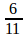b)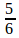c)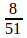d)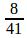e)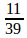Q.5)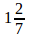th of a number x is 45% of another number y, if the value of y is square of the first two digit even number, what is the difference between x and y?

a) 25

b) 40

c) 55

d) 65

e) 35

Q.6) How many different ways can the letters of the word ‘BUTTERFLY’ be arranged, if the vowels always come together?

a) 42900

b) 41033

c) 40410

d) 40320

e) 41120

Q.7) The speed of the stream is 12 km/hr. The downstream speed is 2x km/hr. The upstream speed is 1x km/hr. Find the speed of the boat in still water.

a) 20 km/hr

b) 24 km/hr

c) 35 km/hr

d) 36 km/hr

e) 38 km/hr

Q.8) A man selling his bike at Rs.33750 he got 25% loss, What price he should sell his bike to get 15% gain?

a) 52780

b) 51750

c) 53780

d) 51340

e) 51220

Q.9) Maha and Mala’s expenditure ratio is 11:13, and their income ratio is 15:18. Maha’s monthly saving is Rs.2000, What is the Mala’s saving?

a) 1200

b) 1800

c) 2100

d) Cannot be determined

e) None of these

Q.10) In a fruit shop, the ratio of sold and unsold apple is 11 : 14, total number of fruits in the shop is 1250, number of apple in the shop is 34%, then number of sold apples is what percentage of number of unsold apples in the shop?

a) 74.76%

b) 75.88%

c) 71.22%

d) 77.65%

e) 78.57%

Q.11) A bag contains 3 white balls, 4 green balls and some yellow balls. If two balls are drawn at random, the probability that two balls are yellow is 1/15. Find the total number of balls in the bag.

a) 9

b) 12

c) 11

d) 14

e) 10

Q.12) The speed of the boat in downstream is 2x km/hr and the speed of the boat in still water is 1.5x km/hr. If the speed of the boat in upstream is 10 km/hr, what is the speed of the stream?

a) 5 km/hr

b) 10 km/hr

c) 15 km/hr

d) 20 km/hr

Q.13) Train A running at the speed of 60 km/hr and passes a platform in 18 seconds. If the length of the train is 200 m, find the length of the platform.

a) 100 m

b) 150 m

c) 200 m

d) 50 m

e) 125 m

D.14-20) Study the pie-chart and answer the questions based on it.

The Pie chart shows the percentage of salary received by five employees from company A.

Total salary is Rs.2.5 lakhsQ.14) If B invested his salary in a bank at the interest rate of 20% at S.I for 3 years, how much interest he received from the bank?

a) Rs.36000

b) Rs.45000

c) Rs.27000

d) Rs.25000

e) Rs.30000

Q.15) What is the ratio of salary received by A and C together to D and F together?

a) 2: 3

b) 3: 4

c) 25: 39

d) 25: 37

e) 23: 36

Q.16) What is the difference between the salary received by B and C together and the salary received by E and F together?

a) Rs.45000

b) Rs.46500

c) Rs.42500

d) Rs.44000

e) Rs.47050

Q.17) If the company reduced 10% of salary for all the five employees, then what is the total salary received by A, C, E and F?

a) Rs.147500

b) Rs.159500

c) Rs.157500

d) Rs.175700

e) Rs.163500

Q.18) What is the total salary received by A and D?

a) Rs.67500

b) Rs.63500

c) Rs.72500

d) Rs.69500

e) Rs.62500

Q.19) The salary received by B is what percentage more than the salary received by D?

a) 50%

b) 45%

c) 55%

d) 40%

e) 60%

Q.20) If the total salary is increased to 3lakhs and the percentage of salary distribution is same, then what is the total salary received by C and D together?

a) Rs.69000

b) Rs.72000

c) Rs.66000

d) Rs.60000

e) None of these

Q.1) e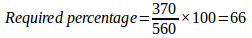Q.2) b

Number of two wheelers sold by all company = 560 + 600 + 430 + 420 + 560 = 2570

Number of four wheelers unsold by all company = 170 + 160 + 180 + 170 + 440 = 1120

Difference = 1450

Q.3) d

Total number of two wheelers manufactured by company U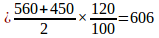Q.4) c

Without replacement,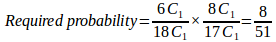Q.5) d

First two digit even number is 10

Then, the value of y = 100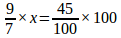X=35

Difference=100 – 35=65

Q.6) d

BTTRFLY(UE)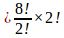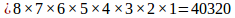Q.7) d

From the question,

12 =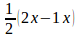X= 24km/hr

Required speed of the boat in still water =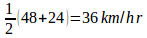Q.8) b

75% = 33750

115%=x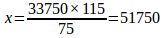Q.9) d

Cannot be determined

Q.10) e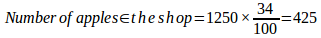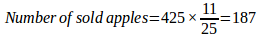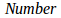of unsold apples = 425 – 187 = 238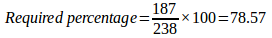Shortcut: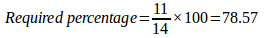Q.11) e

Let number of yellow balls be x

From the question,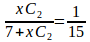X= 3

Total balls = 3+7 = 10

Q.12) a

From the question,

1.5x =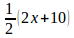X= 10km/hr

Required speed of stream =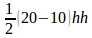=5km/hr

Q.13) a

From the question,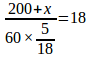X= 100 m

Q.14) c

Salary of B = 18% of 2.5 lakhs= Rs.45000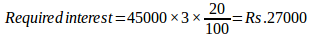Q.15) d

Required ratio = (15%+10%) of 2.5 lakhs: (12% + 25%) of 2.5 lakhs = 25: 37

Q.16) c

Required difference = (20+25)% of 2.5 lakhs- (18+10)% of 2.5 lakhs = 17% of 2.5 lakhs = Rs.42500

Q.17) c

Required total = 90% of (15% + 10% + 20% +25%) of 2.5 lakhs = Rs.157500

Q.18) a

Required total = (15% +12%) of 2.5 lakhs= Rs.67500

Q.19) a

Required % =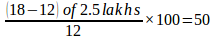Q.20) c

Required total = (10+12)% of 3 lakhs= Rs.66000

We will post this Practice Questions – Daily till the start of the IBPS RRB PO & Assistant (Regularly)

Quantitative Aptitude @ 3:00 P.M

Reasoning Ability -@ 5:00 P.M

Attend GEEK TEST – SOLVE 10 Daily and enhance your preparation to the next level. Attend the Daily Tests with the Timer and improve your solving ability.

Also Please attend this BLUE WHALE QUESTION (which is a combination of many topics and combined into a single one). This is a One Stop Solution for all your Miscellaneous needs.

## BLUE WHALE CHALLENGE QUESTIONS

Get the FULL LENGTH MOCK TEST SERIES for SBI PO Mains 2018 , SBI CLERK Mains 2018 & BOB PO Mains 2018 from our Bankersdaily Store.